Next: Normal equations Up: MULTIVARIATE LEAST SQUARES Previous: MULTIVARIATE LEAST SQUARES

## Inverse filter example

Let us take up a simple example of time-series analysis. Given the input, say, to some filter, say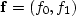, then the output is necessarily. To design an inverse filter, we would wish to have boldc come out as close as possible to (1, 0, 0). So the statement of wishes (17) is(19)
The method of solution is to premultiply by the matrix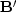, getting(20)
Thus,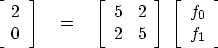(21)
and the inverse filter comes out to be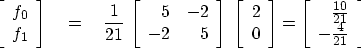(22)
Inserting this value of (f0,f1) back into (19) yields the actual output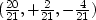,which is not a bad approximation to (1, 0, 0).Next: Normal equations Up: MULTIVARIATE LEAST SQUARES Previous: MULTIVARIATE LEAST SQUARES
Stanford Exploration Project
10/21/1998